C Program to Print Even Numbers in an Array

In this tutorial you will learn about the C Program to Print Even Numbers in an Array and its application with practical example.

C Program to Print Even Numbers in Array

In this tutorial, we will learn to create a C program that will Print Even Numbers in Array using C programming.

Prerequisites

Before starting with this tutorial we assume that you are best aware of the following C programming topics:

• Operators in C Programming.
• Basic Input and Output function in C Programming.
• Basic C programming.
• For loop in C programming.
• Conditional Statements in C programming.
• Arithmetic operations in C Programming.

Program to Print Even Numbers in Array

In c programming, it is possible to take numerical input from the user and Print Even Numbers in Array with the help of a very small amount of code. The C language has many types of header libraries which has supported function in them with the help of these files the programming is easy.

The Even numbers are those numbers that are completely divisible by 2. which means the reminder should be zero.

With the help of this program, we can Print Even Numbers in Array.

Output:-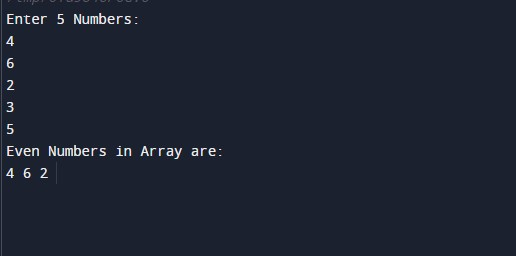In the above program, we have first initialized the required variable.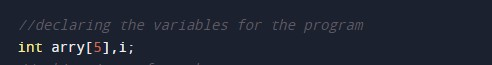• arry= it will hold the integer value of the input.
• i = it will hold the integer value.

Input message for the user for five integer values.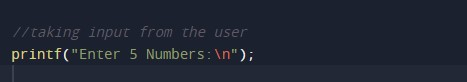Input for loop for taking array elements.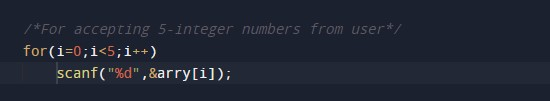Program Logic Code.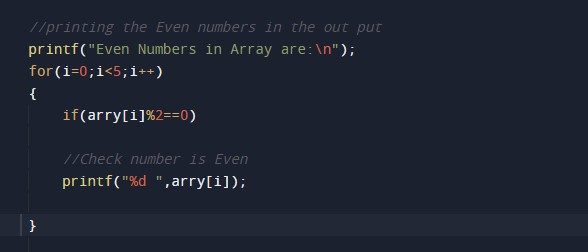Printing output Even digits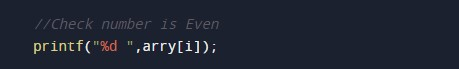In this tutorial we have learn about the C Program to Print Even Numbers in an Array and its application with practical example. I hope you will like this tutorial.